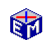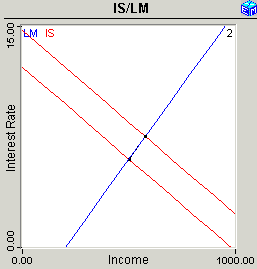The Keynesian IS/LM Model

The Keynesian IS/LM Model explains how the economy can be in equilibrium even with unemployment in the labor market.  There are two versions of this story in this application.Model Link:  IS/LM Basics
<
Printable PDF ExercisesThe IS/LM Basics application shows you how to derive the IS and LM curves and how to do the basic short-run analysis of monetary and fiscal policy assuming a fixed price level.  The steps involved are:

• Derive IS Curve
• Derive LM Curve
• Monetary Policy
• Fiscal Policy (See the EconModel instructions to the right and one of the resulting graphics below.)

The following movie is a brief excerpt from the IS/LM Basics application.

Movie:  Basic Monetary and Fiscal Policy Analysis
(2 min, 43 seconds)

The Aggregate Supply / Aggregate Demand extension adds to the analysis an AS/AD diagram that accommodates analysis of a flexible price level.  You can then trace out the short-run and long-run implications of monetary and fiscal policy.Model Link:  Aggregate Supply / Aggregate Demand
<
Printable PDF Exercises

The steps involved are:

• Derive Aggregate Demand Curve
• Fiscal Policy, Short-Run and Long-Run
• Monetary Policy, Short-Run and Long-Run

You might also be interested in the Animated Phillips Curve Diagram.  The original pre-1969 Phillips Curve appeared to support the idea that there was a stable tradeoff between inflation and unemployment, and this stylized fact was taken to support a Keynesian view of the economy.

Classic Economic Models

Macroeconomics

Introduction
Overview of Macro Models

Microeconomics

Introduction
Overview of Micro Models

Resources